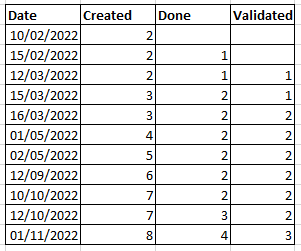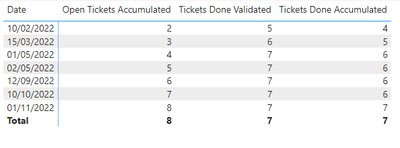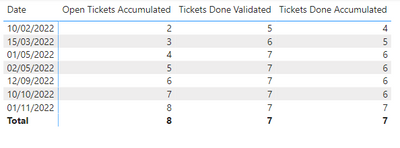cancel
Showing results for
Did you mean:Helper I

## Running Total by date counting rows

I have two tables:

Table 1:  Calendar table. The calendar table is linked to the tickets table with the

Table 2:  Tickets table  called “Jira” as belowI want to obtain a running table that will look like:It will give the running accumulate of the tickets created, those with Date done, and those with Date Validated

With this formula I was able to get the running total of the Created one, but I am not able to get the other two

Open Tickets Accumulated = CALCULATE(

COUNTROWS(Jira),

FILTER(

ALL(Calendar[Date]),

Calendar[Date] <= MAX(Jira[Date Created])

)

)

Can you help me?

3 REPLIES 3Helper I

It is not working as expected.  this is what I am gettingAt the same time as I will like to filter by name, for example if I filter by Abel I am gettingWhich is what I am expecting

What do I need to update?Super User

@vzbkb1 Try:

``````Tickets Done Accumulated =
VAR __Date = MAX('Jira'[Date Created])
VAR __Table = FILTER(ALL('Jira'), [Date Done] < __Date)
VAR __Result = COUNTROWS(__Table)
RETURN
__Result

Tickets Done Validated =
VAR __Date = MAX('Jira'[Date Created])
VAR __Table = FILTER(ALL('Jira'), [Date Validated] < __Date)
VAR __Result = COUNTROWS(__Table)
RETURN
__Result
``````

Become an expert!: Enterprise DNA
External Tools: MSHGQM
Latest book!:
Mastering Power BI 2nd EditionDAX is easy, CALCULATE makes DAX hard...Helper I

It is not working as expected.  this is what I am gettingAt the same time as I will like to filter by name, for example if I filter by Abel I am gettingWhich is what I am expecting

What do I need to update?